#### 2021-09-07

Full documentation and tutorials can be found on the isobxr website.

The sweep_steady function is designed to allow the user to map the combined effects of two parameters over the final state of an isobxr box model.

The sweep_steady workflow can be found here.

The sweep_steady function is built as a 2 steps composite scenario.

1. The first run (run #1) is used to force the initial conditions of the second run. It is run once only.

2. The second run (run #2) is repeated for all the combinations of the two parameters values.
• Each parameter varies over a range of values defined by the user:
• parameter #1 is ranging over n values, parameter #2 over m values.
• Run #2 is repeated over the whole 2D space of parameters ($$n \times m$$ times).

The main purpose of this sweep_steady structure is:

• to explore the influence of a pair of parameters over the distribution of isotopes in the system at the final state of run #2
• while starting this run #2 from a common initial state, defined by run #1
• run #1 typically being for a balanced system, relaxed to it's steady state

# Sweep steady master file (xlsx)

In addition to the global isobxr master file, the sweep_steady function requires a sweep steady master file.

The sweep steady master file is the (xlsx) document containing all commands allowing sweep_steady function to compose a scenario of 2 runs.

The two parameters to be swept over run #2 are defined by user directly in the function input, in the R console.

The format of the sweep steady master file is the same as the compose master file and the sweep dynamic master file.

It is where the user sets the design of their 2 runs scenario to be swept:

• necessary: define the list of 2 runs composing the scenario (run durations, run resolutions, lists of fluxes, lists of coefficients).
• optional: define the forcings over one or both runs composing the composite scenario (FORCING_RAYLEIGH, FORCING_ALPHA, FORCING_DELTA, FORCING_SIZE).

The format of the sweep steady master file is standardized. The user is encouraged to comply with these standards, as described thereafter, otherwise there is a high probability for these functions to crash.

### File name and Location

• The sweep steady master file is an xlsx file.
• The sweep steady master file name is chosen by user and specified in the sweep_steady function inputs: [e.g., 0_SWEEP_STEADY_MASTER.xlsx ].
• the sweep steady master file should be stored in the directory in which isobxr master file is found, where all runs for this box model system will be performed and where all outputs will be stored in automatically created subdirectories. This directory corresponds to the working directory (workdir) used as parameter of the sweep_steady function.

### File structure

The sweep steady master file contains the 5 following sheet strictly named as follows:

1. RUN_LIST: define the list of 2 runs composing the sweep steady scenario (run durations, run resolutions, lists of fluxes, lists of coefficients).
2. FORCING_RAYLEIGH: define Rayleigh forcings over one or several runs composing the sweep 2 steps scenario.
3. FORCING_ALPHA: define Rayleigh forcings over one or several runs composing the sweep 2 steps scenario.
4. FORCING_DELTA: define initial delta forcings over one or several runs composing the sweep 2 steps scenario.
5. FORCING_SIZE: define initial box size forcings over one or several runs composing the sweep 2 steps scenario.

### RUN_LIST sheet (REQUIRED)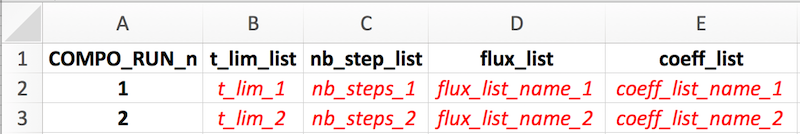The sweep_steady function strictly requires a list of 2 successive runs.

Both of these runs should be successively described one by one on two rows of this sheet, with notably:

1. the run number (COMPO_RUN_n column)
2. the run duration (t_lim_list column)
3. the run resolution (nb_steps_list)
4. the flux list name (flux_list) that will be read from isobxr master file
5. the coefficients list name (coeff_list) that will be read from isobxr master file

### FORCING_SIZE sheet (OPT)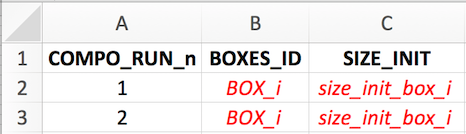See composite master file format description for details.

### FORCING_DELTA sheet (OPT)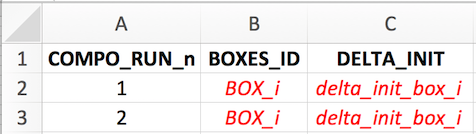See composite master file format description for details.

### FORCING_ALPHA sheet (OPT)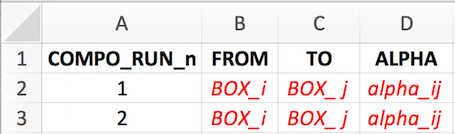See composite master file format description for details.

### FORCING_RAYLEIGH sheet (OPT)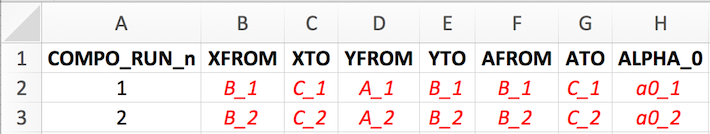See composite master file format description for details.

The user needs to define the two runs composing the 2 steps scenario.

1. The first run is used to let the system relax to its steady state used as an initial state in run #2.
1. The user should consider here a balanced system. The isobxr functions will thus use the analytical solver (ana_slvr).
2. We thus recommend the user to set the number of steps for run #1 (nb_steps) to 1 (its' minimum value).
This allows to only calculate the final state of the system, at the end of run #1.
This reduces the calculation time and data produced without affecting the accuracy of the calculation.
2. The second run is used to explore the final state of the system as a function of all combinations of parameters #1 and #2 values.
1. The main purpose of this function is to explore the influence of the 2 combined parameters over the final state of a system that relaxed to it's steady state.
2. It is therefore recommended to use the same balanced system over the second run, allowing to analytically solve the system (with ana_slvr).
3. The use of the analytical solver will notably allow to map the final state at a high resolution of parameters #1 and #2
while minimizing the required calculation time as well as RAM and hard memory.
4. We thus recommend the user to set the number of steps for run #2 (nb_steps) to 1 (its' minimum value).
This allows to only calculate the final state of the system, at the end of run #2.
This reduces the calculation time and data produced without affecting the accuracy of the calculation.
remark
The reason why the sweep_steady function begins with a run #1 which final state defines a common initial state for all repeated run #2 sweeps is that it can allow the user to sweep the final state of a system in the course transient/dynamic state of run #2, being in a balanced or unbalanced system. This needs however to be designed with care as depending on the defined or forced parameters on run #2 vs. run #1, the continuity of the conditions of run #1 and run #2 is not certain. It is then advised to use the better suited sweep_dyn function described thereafter.
Forcings
The user can define some forcings over the system (will affect both run #1 and run #2).
These forcings will overwrite the conditions set by the reading of the isobxr master file.
These forcings will be overwritten by the swept parameters that affect run #2.

## Required arguments

In addition to all the usual input parameters required for the sweep_steady function, the user has to define the two parameters to be swept.

There are 6 types of sweepable parameters (or that can be explored), which names are strictly defined as follows:

#>  "EXPLO_n_FLUX_MATRICES"
#>  "EXPLO_n_ALPHA_MATRICES"
#>  "EXPLO_1_SIZE"
#>  "EXPLO_1_DELTA"
#>  "EXPLO_1_ALPHA"
#>  "EXPLO_1_RAYLEIGH_ALPHA"

### EXPLO_n_FLUX_MATRICES

This type of parameter allows to explore a series of flux lists as defined in isobxr master file.

data.frame(VALUES = c("flux_list_1",  # vector of n strings of characters
"...",
"flux_list_i",
"...",
"flux_list_n"),
EXPLO_TYPES = "EXPLO_n_FLUX_MATRICES") # stricly leave as such

The EXPLO_n_FLUX_MATRICES parameter will allow the sweep_steady function to sweep the effect of a series of flux lists (defining flux matrices and initial box sizes) on run #2 evolution.

The format of this data frame should be exactly as shown above.

The values are a vector of strings of characters containing the list of flux list names, that will be called from the isobxr master file

### EXPLO_n_ALPHA_MATRICES

This type of parameter allows to explore a series of lists of coefficients as defined in isobxr master file.

data.frame(VALUES = c("coeff_list_1", # vector of n strings of characters
"...",
"coeff_list_i",
"...",
"coeff_list_n"),
EXPLO_TYPES = "EXPLO_n_ALPHA_MATRICES") # stricly leave as such

The EXPLO_n_ALPHA_MATRICES parameter will allow the sweep_steady function to sweep the effect of a series of isotope fractionation coefficient lists (defining coefficient matrices) on run #2 evolution.

The format of this data frame should be exactly as shown above.

The values are a vector of strings of characters containing the list of coefficients list names, that will be called from the isobxr master file

### EXPLO_1_SIZE

This type of parameter allows to explore a range of sizes for a given box.

data.frame(BOXES_ID = "BOX_i", # 1 string of char
SIZE_MIN = "min_sweep_value", # 1 numerical value
SIZE_MAX = "max_sweep_value", # 1 numerical value
SIZE_STEPS = "sweep_steps", # 1 numerical value
EXPLO_TYPES = "EXPLO_1_SIZE") # stricly leave as such

The EXPLO_1_SIZE parameter will allow the sweep_steady function to sweep the effect of a range of box sizes for a given box on run #2 evolution.

The format of this data frame should be exactly as shown above.

### EXPLO_1_DELTA

This type of parameter allows to explore a range of delta values for a given box.

data.frame(BOXES_ID = "BOX_i", # 1 string of char
DELTA_MIN = "min_sweep_value", # 1 numerical value
DELTA_MAX = "max_sweep_value", # 1 numerical value
DELTA_STEPS = "sweep_steps", # 1 numerical value
EXPLO_TYPES = "EXPLO_1_DELTA") # stricly leave as such

### EXPLO_1_ALPHA:

This type of parameter allows to explore a range of alpha (coeff) values for a given flux.

data.frame(FROM = "BOX_i", # 1 string of char
TO = "BOX_j", # 1 string of char
ALPHA_MIN = "min_sweep_value", # 1 numerical value
ALPHA_MAX = "max_sweep_value", # 1 numerical value
ALPHA_STEPS = "sweep_steps", # 1 numerical value
EXPLO_TYPES = "EXPLO_1_ALPHA") # stricly leave as such

### EXPLO_1_RAYLEIGH_ALPHA:

This type of parameter allows to explore a range of incremental alpha values for a Rayleigh distillation model.

data.frame(XFROM = "Box_B", # B>C flux at numerator (strings of char)
XTO = "Box_C",
YFROM = "Box_A", # A>B flux at denominator (strings of char)
YTO = "Box_B",
AFROM = "Box_B", # resulting fract. coefficient (strings of char)
ATO = "Box_C",
ALPHA_0_MIN = "min_sweep_value", # value of incremental B>A coeff. (num.)
ALPHA_0_MAX = "max_sweep_value",
ALPHA_0_STEPS = "sweep_steps",
EXPLO_TYPES = "EXPLO_1_RAYLEIGH_ALPHA") # stricly leave as such

## Default and optional outputs

By default, the sweep_steady prints in the R session a series of default heatmap plots of the delta values of each system finite boxes at the final state of the run in the 2D space of parameters.

All sweep_steady output data is stored in a temporary directory by default.

The user can save these outputs to their working directory by changing the save_run_outputs argument to TRUE.

The sweep_steady outputs are structured as shown in the full package documentation.

By default, the sweep_steady prints in the R session a series of default heatmap plots of the delta values of each system finite boxes at the final state of the run in the 2D space of parameters.

The outputs of the sweep_steady can be plotted using the shinobxr_app function.

The shinobxr_app function launches an interactive html app allowing to edit and export graphical representation of the model runs.

The shinobxr_app requires the user to save the sweep_steady output files to a local working directory (by setting the argument save_run_outputs = TRUE).

More on the use of the shinobxr_app function can be found on the Shiny app page.

# Tutorial example

Sweeping two fractionation coefficients in a 3-boxes balanced open system.

Here:

• we perform a sweep of the $$\alpha _{C \to B}$$ values and $$\alpha _{A \to C}$$ values at steady state of run #2
• starting from a run #1 at steady state obtained by the relaxation of the system with the a1 coefficients list and the Fx3_ABC_open_bal flux list

No forcings are applied.

To do so, the sweep_steady function can be called as follows:

sweep_steady(workdir = "/Users/username/Documents/1_ABC_tutorial",
# name of the series ID
# in/out time units for pdf plots
time_units =  c("d", "yr"),
# name of sweep std master file
EXPLO_MASTER = "0_SWEEP_STD_MASTER.xlsx",
# make alpha C to B vary between 1 and 1.0005
EXPLO_AXIS_1 = data.frame(FROM = c("C"),
TO = c("B"),
ALPHA_MIN = 1,
ALPHA_MAX = 1.0005,
ALPHA_STEPS = 0.00005,
EXPLO_TYPES = "EXPLO_1_ALPHA"),
# make alpha A to C vary between 0.9996 and 1
EXPLO_AXIS_2 = data.frame(FROM = c("A"),
TO = c("C"),
ALPHA_MIN = 0.9996,
ALPHA_MAX = 1,
ALPHA_STEPS = 0.00005,
EXPLO_TYPES = "EXPLO_1_ALPHA"),
to_STD_DIGEST_CSVs = TRUE)

The sweep_steady will prompt a question to user checking if the number of iterative runs is validated. The higher this number is the longer the run is.

This run will produce a series of default heatmap plots of the delta values of each system finite boxes at the final state of the run in the 2D space of parameters.

You can explore the outputs by then using the isobxr Shiny app (shinobxr_app function, presented below).